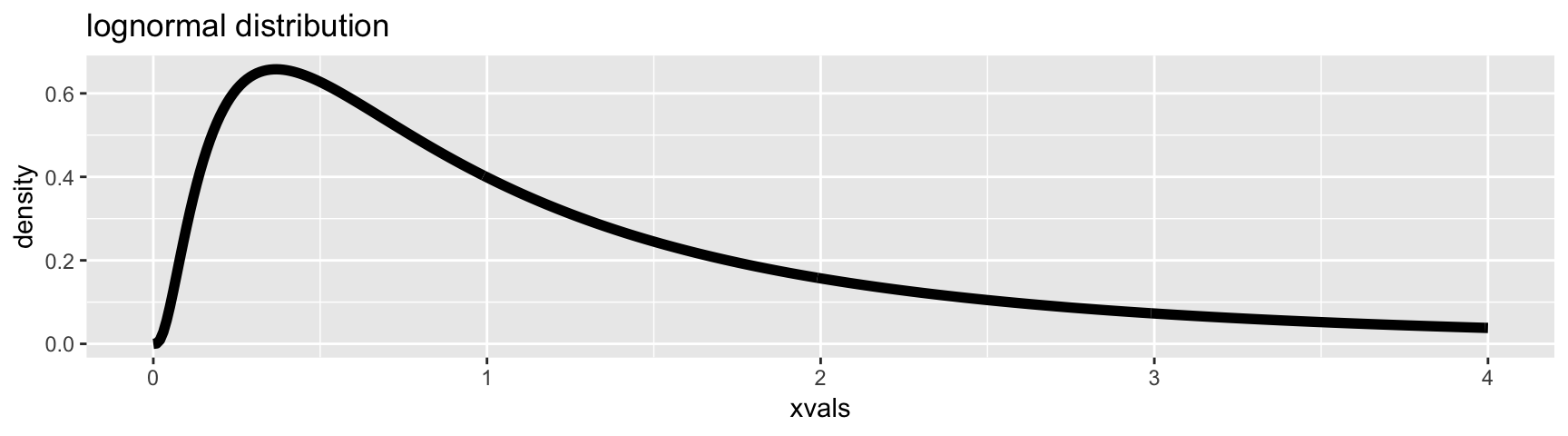## Random Variables

A random variable –in statistical terms– is a variable whose value depends on random chance.

Each random variable has one or more parameters governing the probability of different outcomes.

There are two types of random variables:

discrete random variables which have a limited number of possible discrete outcomes and

continuous random variables which have a (theoretically) unlimited number of possible outcomes.

Think of two examples of a discrete random variables and two examples of a continuous random variables for your area of scientific interest. What is the sample space for the random variable?

## Probability Distributions

If we plot the values from a random variable using a histogram, the shape of the histogram reflects the shape of the probability distribution of that random variable.

The various distributions form the basis of parametric statistics.

## Binomial Distribution

A Bernouili trial describes an event that has exactly two possible outcomes: success and failure.

Success occurs with a given probability, for example $$p = 0.2$$.

We know how likely a trial is to result in success, but any given trial may result in either success or failure, and this cannot be predicted for a single trial (the outcome is stochastic).

The result of a series of $$n$$ Bernoulili trials with $$X$$ successful outcomes results in a binomial random variable.

The expected value of the binomial distribution is $$E(x) = np$$

## Binomial Distribution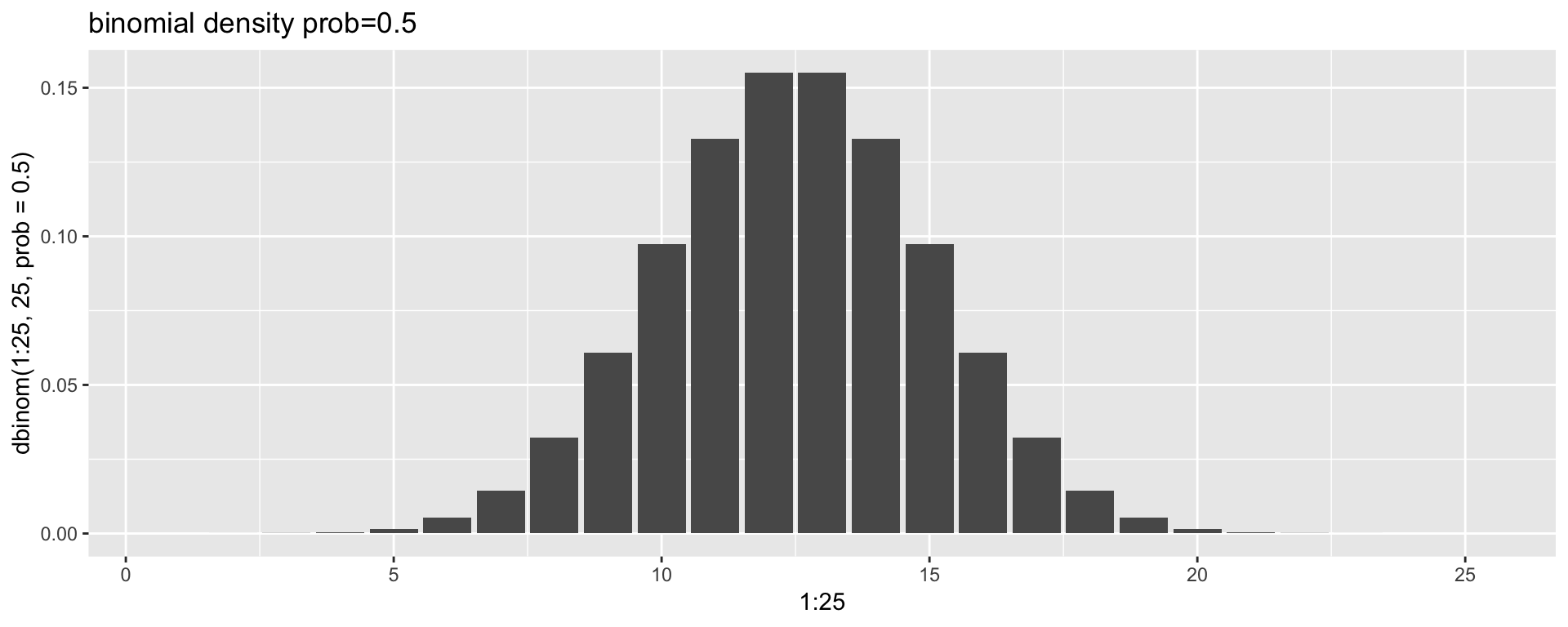## Binomial Distribution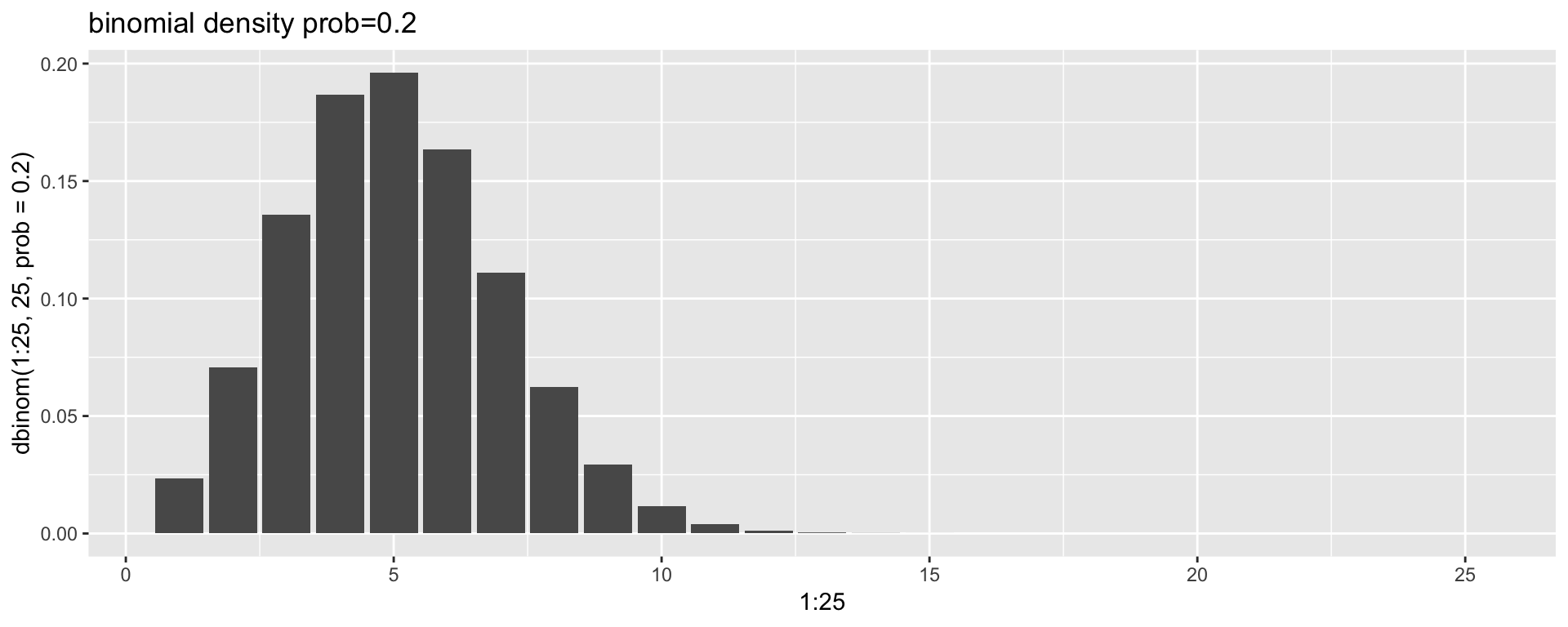## Poisson Distribution

Similar to the binomial distribution, but describes rare events, when the number of trials $$n$$ is unknown.

Requires a single rate parameter $$\lambda$$.

It is commonly pops up when examining the number of events occuring through time (e.g., the number of pieces of mail recieved per day, or the number of speciation events occuring per millenium).

The expected value and variance for the Poisson distribution are both equal to lambda

## Poisson Distribution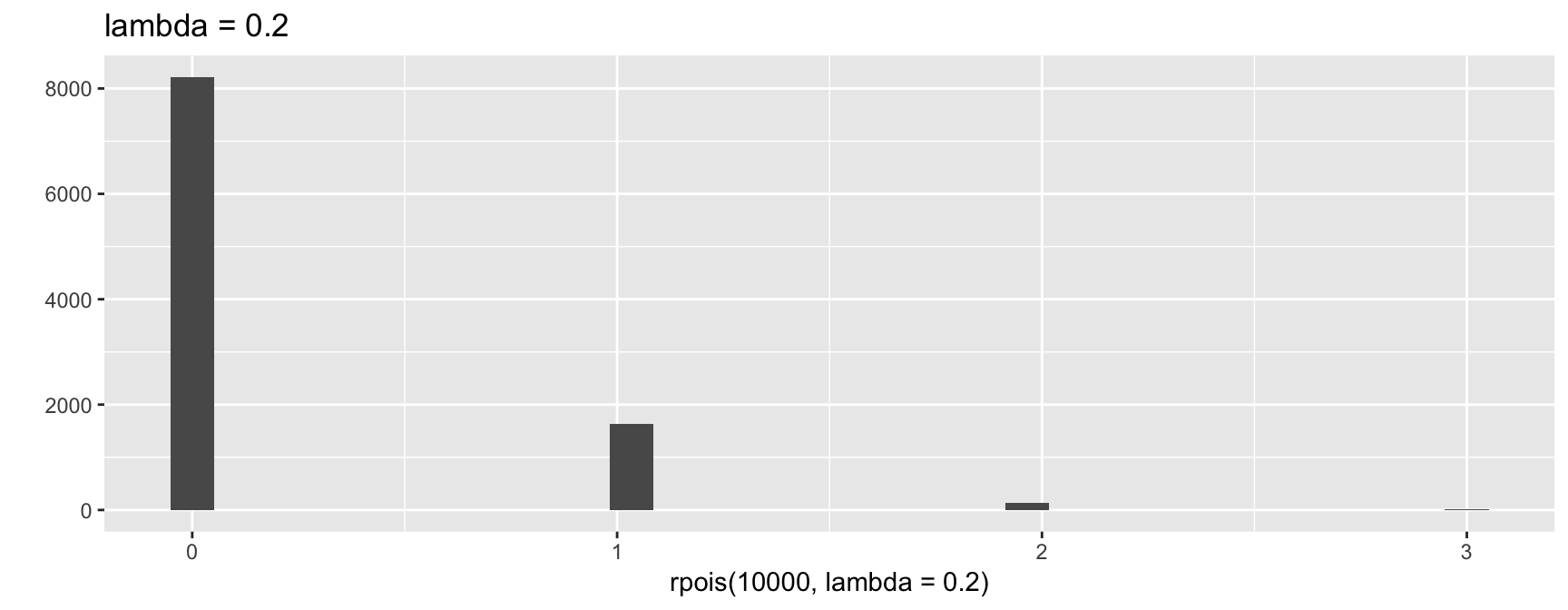## Poisson Distribution

At large values of lambda, the Poisson approximates a normal distribution with mean $$\lambda$$.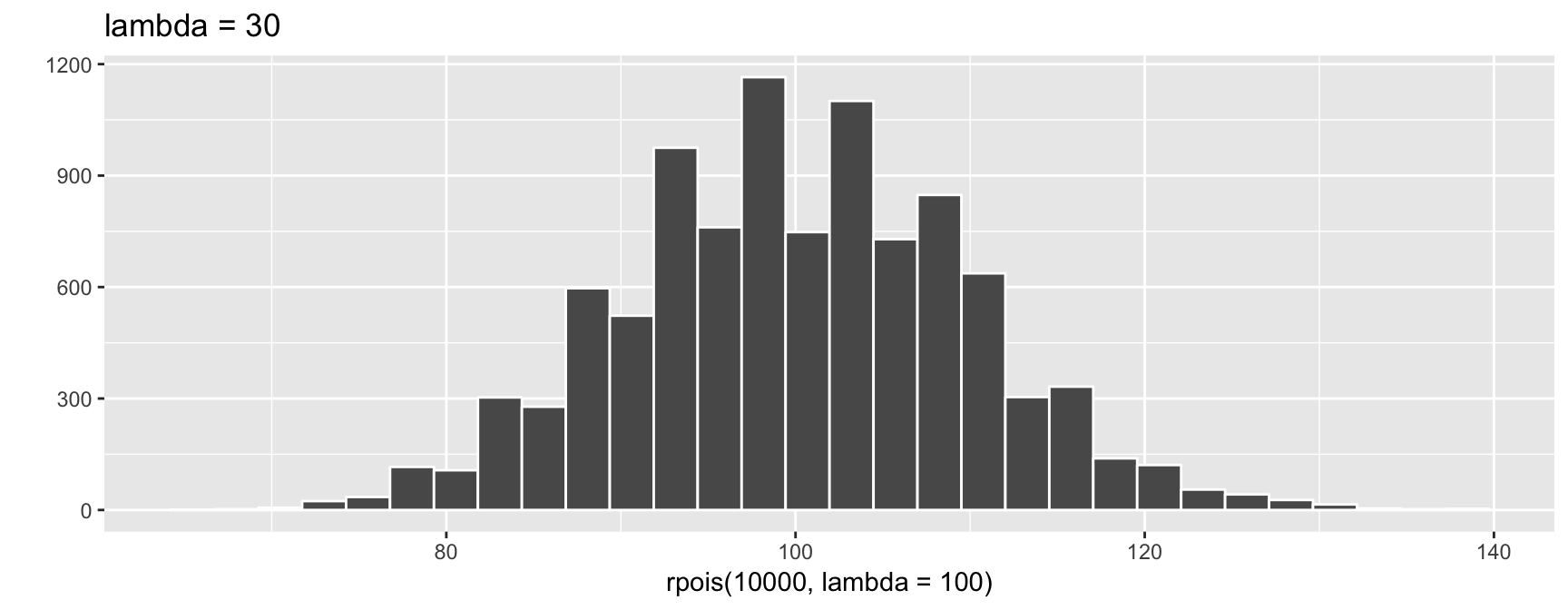## Uniform Distribution

The uniform distribution represents a function in which the probability density is equal for each sub-interval across the a given range.

This results in a flat frequency distribution.

The expected value over the range $$a$$ to $$b$$ is $$(a + b)/2$$.

An example might be the spatial coordinates of plants which are competing for nutrients and light.

## Uniform Distribution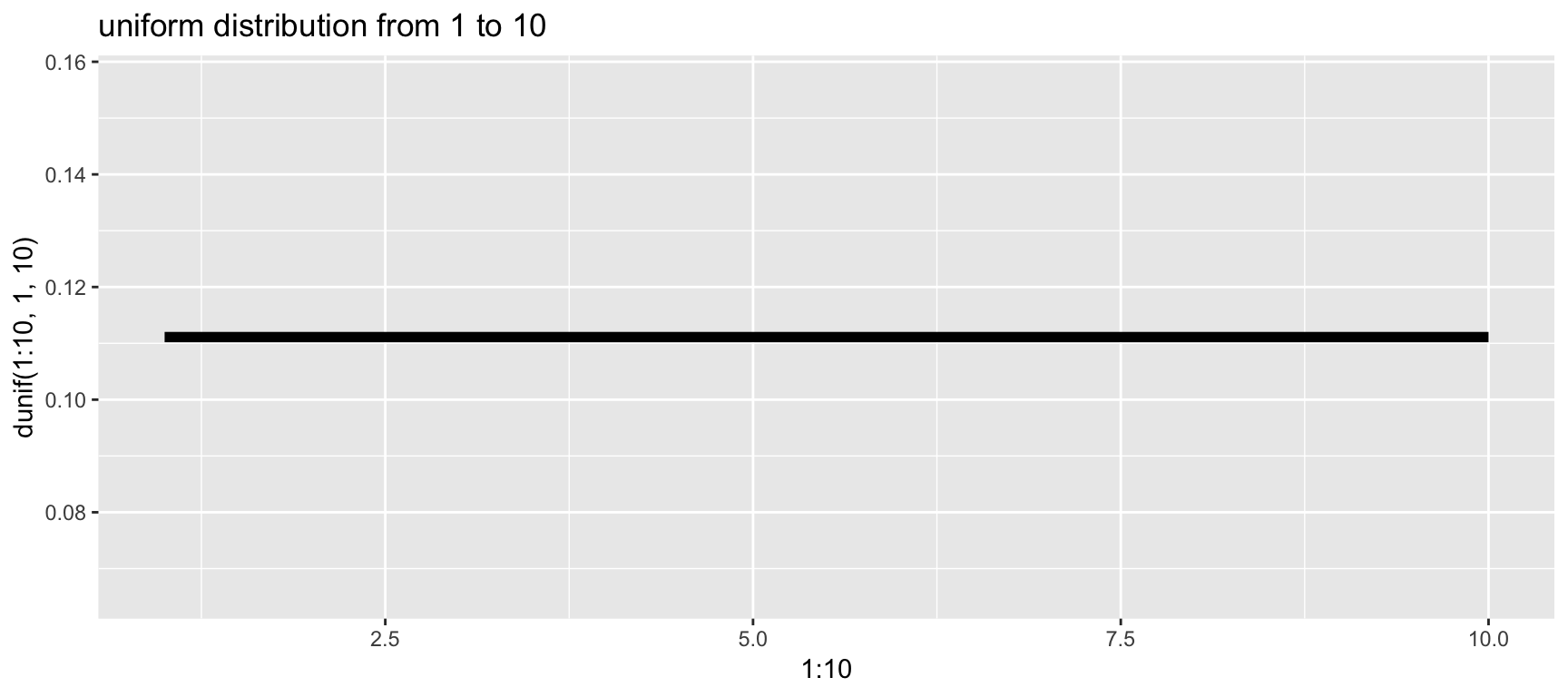## Normal Distribution

The normal distribution (or Gaussian distribution) is the familiar bell-curve shaped distribution that is symmetrical around the mean, with diminishing tails.

Many phenomena in nature are distributed as a normal distribution, especially continuous measurement values.

The normal distribution has two parameters, the mean ($$\mu$$) and the standard deviation ($$\sigma$$).

## Normal Distribution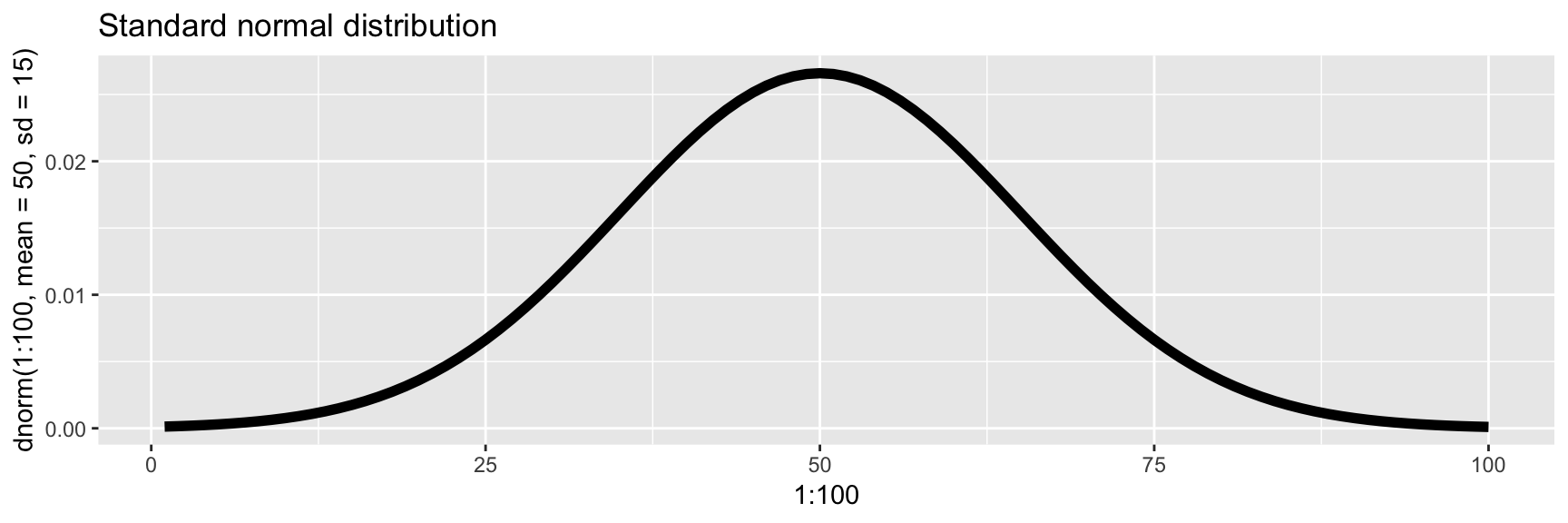## Log-normal Distribution

The log-normal distribution resembles a normal distribution when it is logged.